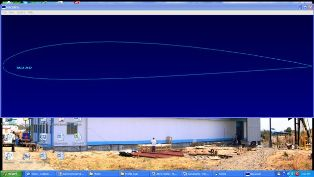Welcome to Aerotech Energy PVT. Ltd

Support +91 22 28965916

FRP FANSImpeller Design

Practical Impeller Design:

This idealized treatment is only a starting point. Further factors we consider while designing include the following:

1. The air will leave the impeller at the angle set by the trailing edge of the blade. There will be a deviation angle tending to reduce input and output power. This is a function of aerofoil shape and blade loading.
2. The air will meet the leading edge of the blade at the optimum angle only at the design volume flow. At other flows the incidence angle will change. The effect of this change on performance over the whole fan characteristics is again a function of aerofoil shape and loading.
3. To keep the portion of flow considered between the imaginary surfaces defining its intended path requires a balancing transverse pressure gradient. In the axial case, for example, only the so-called free vortex pressure and velocity distribution will secure flow at constant radius along concentric cylinders. This distribution requires a constant values of rv cos a, i.e. a spin components which is smaller the bigger the radius. This limits the workdone towards the blade tips, giving a fan of poor power-size ratio; To overcome this weakness, forced vortex designs are used, increasing tip work. To maintain balance the streamlines tilt outwards through the impeller, so that r2and u2 are greater than r1 and u1.
4. Viscous drag forces at the blade surface and wake effects behind the blade convert some of the work input into heat instead of useful pressure rise.
5. Tip clearance effects and boundary layer retorted flow along casing hub, back plate spoils the flow pattern at the ends of the blade and limits the work done (input and output).
6. The velocity leaving the blades is usually far from uniform in magnitude or direction. Since the energy is proportional to the square of the velocity, more is required by the peak velocities than is saved in the troughs. Thus excess kinetic energy is supplied which not all will be available when the air has reached the downstream test plane.

On account of the considerable variation in the flow conditions and the blade section along the span, it is divided in to a number of infinitesimal section of small radial thickness dr. the flow through such a section is assumed to be independent of the flow through other elements.

Velocities is a blade force for the flow through the elemental section are shown below. The flow has a mean velocity w and direction b (from the axial direction). The lift force L is normal of mean flow and the drag D parallel to this. The axial (#Fx) and tangential (#Fy ) force acting on the element. Also (#Fr) is the resultant force inclined at an angle 0 to the direction of lift.

An expression for the pressure rise (#P) across the element is now developed.

Resolving the force in the axial and tangential direction,

#Fx = #L sinb - #D cosb

#Fy = #L cosb + #D sin b

By definition lift and drag force are

#L = ½ CL p w2 (ldr)

#D = ½ CD p w2 (ldr)

tanf = #D/#L = CD/CLPERFORMANCE COEFFICIENTS:

Since the fan laws are valid for any particular point on the fan pressure/volume characteristic, similar laws will be valid for every other point of operation, the only difference being numerical values of the coefficients. Thus a plot of Kp against Kq will have exactly same form as the pressure /volume characteristic of each fan in homologous series, and also be used to compare the performance of the series design with that of another series design. A system of performance coefficients based on this;

 Volume coefficient.       Kq Q  = ----------------------------                d3 (n / 1000) Pressure coefficient.       Kr Fan total pressure Pt   = -------------------------------              d2 (n / 1000)2 Fan power efficient.       Kp Fan h.p.  = -------------------------------              d5 (n / 1000)3 Volume coefficient        f Volume flow  = --------------------------------                (Pd2/ 4)u Total pressure coefficient   y Fan total pressure  = -----------------------------                  ½pu2 Static pressure coefficient    yst Fan static pressure   = ------------------------------                  ½pu2 Power coefficient. l fy  = -------------------------------                        h

Application of Fan Engineering Formula:

The application of the fan theory can best shown by example.

 The application of the fan theory can best shown by example. D =      ÖQ/k Hub Dia. =   Tpf/n x 10 RPM =   (U)/(p x d x 60) Stp =   0.5 x p x ts2 (1- f αtan2θ) Tip Speed =   p x D x n a =   U/cx Tp =   Stp + Pv Fan Velocity pressure (Vp) =   0.5 x r x u2 Required Impeller pressure =   Tp/h Impeller Power =   Fp/Gh/Mh Aerodynamic efficiency =   Ap/Ip Tip Clearance =   D x 0.025 x 25.4 Mass flow rate =   r x Q Natural frequency =   1/2p Ög´ 1000                 d Blade pass Frequency =   (n x Z )/60 Frequency of natural vibration =   1Ög      2*p d Solid ratio =   ( Z x C)/2 pr Lift force =   0.5 x p x V 2 x S x CL Drag force =   0.5 x p x V 2 x S x CD Axial thrust =   5.202 x D r x NFA2 x W Centrifugal force =   2 x p x N_         60   x 2 x p x M `                           r Reynolds number =   DVr        m Fan efficiency =     Q x tp       x (100)       kW x 1000

OUR CLIENTS